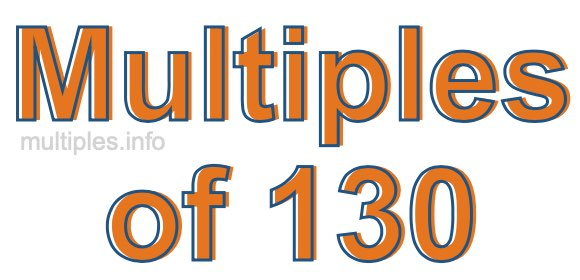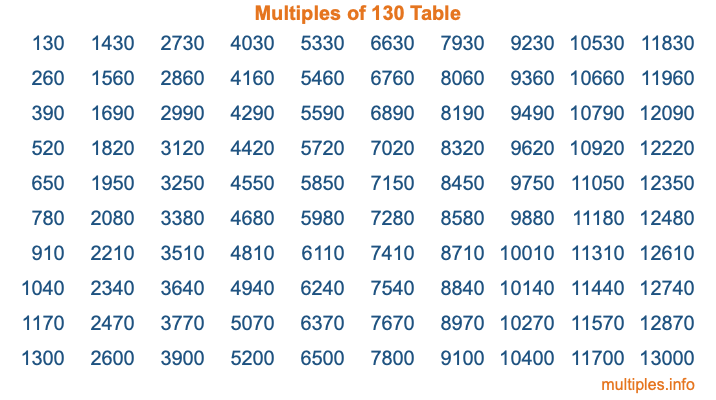Multiples of 130Welcome to the Multiples of 130 page. Here we will first teach you everything you will ever need to know about the multiples of 130, and then give you a study guide summary of everything we taught you to make sure you remember it all. Use this page to look up facts and learn information about the multiples of 130. This page will make you a multiples of one hundred thirty expert!

Definition of Multiples of 130
Multiples of 130 are all the numbers that when divided by 130 equal an integer. Each of the multiples of 130 are called a multiple. A multiple of 130 is created by multiplying 130 by an integer.

Therefore, to create a list of multiples of 130, you start with 1 multiplied by 130, then 2 multiplied by 130, then 3 multiplied by 130, and so on for as long as you want. Thus, the list of the first five multiples of 130 is 130, 260, 390, 520, and 650. To see a larger list of multiples of 130, see the printable image of Multiples of 130 further down on this page. We also have a category where you can choose any nth multiple of 130.

Multiples of 130 Checker
The Multiples of 130 Checker below checks to see if any number of your choice is a multiple of 130. In other words, it checks to see if there is any number (integer) that when multiplied by 130 will equal your number. To do that, we divide your number by 130. If the the quotient is an integer, then your number is a multiple of 130.

Is  a multiple of 130?

Least Common Multiple of 130 and ...
A Least Common Multiple (LCM) is the lowest multiple that two or more numbers have in common. This is also called the smallest common multiple or lowest common multiple and is useful to know when you are adding our subtracting fractions. Enter one or more numbers below (130 is already entered) to find the LCM.

Check out our LCM Calculator if you need more details about the Least Common Multiple or if you need the LCM for different numbers for adding and subtraction fractions.

nth Multiple of 130
As we stated above, 130 is the first multiple of 130, 260 is the second multiple of 130, 390 is the third multiple of 130, and so on. Enter a number below to find the nth multiple of 130.

th multiple of 130

Multiples of 130 vs Factors of 130
130 is a multiple of 130 and a factor of 130, but that is where the similarities end. All postive multiples of 130 are 130 or greater than 130. All positive factors of 130 are 130 or less than 130.

Below is the beginning list of multiples of 130 and the factors of 130 so you can compare:

Multiples of 130: 130, 260, 390, 520, 650, etc.

Factors of 130: 1, 2, 5, 10, 13, 26, 65, 130

As you can see, the multiples of 130 are all the numbers that you can divide by 130 to get a whole number. The factors of 130, on the other hand, are all the whole numbers that you can multiply by another whole number to get 130.

It's also interesting to note that if a number (x) is a factor of 130, then 130 will also be a multiple of that number (x).

Multiples of 130 vs Divisors of 130
The divisors of 130 are all the integers that 130 can be divided by evenly. Below is a list of the divisors of 130.

Divisors of 130: 1, 2, 5, 10, 13, 26, 65, 130

The interesting thing to note here is that if you take any multiple of 130 and divide it by a divisor of 130, you will see that the quotient is an integer.

Multiples of 130 Table
Below is an image of the first 100 multiples of 130 in a table. The table is in chronological order, column by column. The first column has the first ten multiples of 130, the second column has the next ten multiples of 130, and so on.The Multiples of 130 Table is also referred to as the 130 Times Table or Times Table of 130. You are welcome to print out our table for your studies.

Negative Multiples of 130
Although not often discussed or needed in math, it is worth mentioning that you can make a list of negative multiples of 130 by multiplying 130 by -1, then by -2, then by -3, and so on, to get the following list of negative multiples of 130:

-130, -260, -390, -520, -650, etc.

Multiples of 130 Summary
Below is a summary of important Multiples of 130 facts that we have discussed on this page. To retain the knowledge on this page, we recommend that you read through the summary and explain to yourself or a study partner why they hold true.

There are an infinite number of multiples of 130.

A multiple of 130 divided by 130 will equal a whole number.

130 divided by a factor of 130 equals a divisor of 130.

The nth multiple of 130 is n times 130.

The largest factor of 130 is equal to the first positive multiple of 130.

130 is a multiple of every factor of 130.

130 is a multiple of 130.

A multiple of 130 divided by a divisor of 130 equals an integer.

130 divided by a divisor of 130 equals a factor of 130.

Any integer times 130 will equal a multiple of 130.

Multiples of a Number
Here you can get the multiples of another number, all with the same attention to detail as we did for multiples of 130 on this page.

Multiples of
Multiples of 131
Did you find our page about multiples of one hundred thirty educational? Do you want more knowledge? Check out the multiples of the next number on our list!